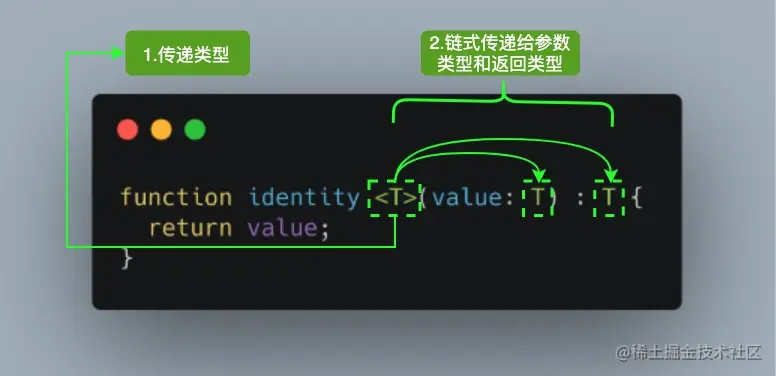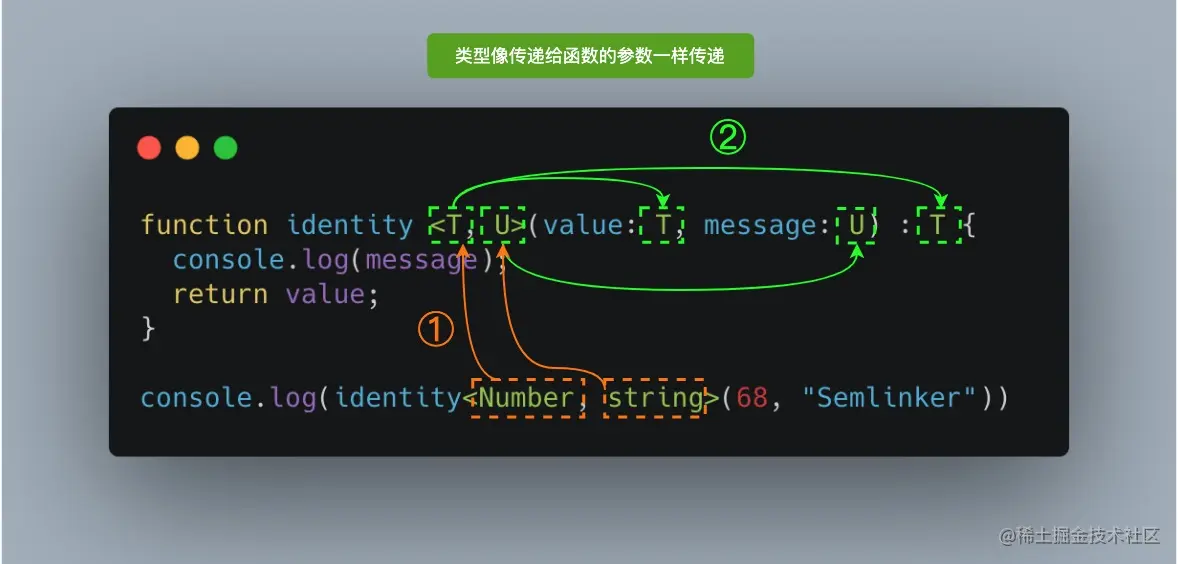# 细数这些年被困扰过的 TS 问题

14,840

TypeScript 是一种由微软开发的自由和开源的编程语言。它是 JavaScript 的一个超集，而且本质上向这个语言添加了可选的静态类型和基于类的面向对象编程。

TypeScript 提供最新的和不断发展的 JavaScript 特性，包括那些来自 2015 年的 ECMAScript 和未来的提案中的特性，比如异步功能和 Decorators，以帮助建立健壮的组件。

### 一、如何在 window 对象上显式设置属性

``````Property 'MyNamespace' does not exist on type 'Window & typeof globalThis'.(2339)
``````

``````(window as any).MyNamespace = {};
``````

``````declare interface Window {
MyNamespace: any;
}

window.MyNamespace = window.MyNamespace || {};
``````

``````/**
* A window containing a DOM document; the document property
* points to the DOM document loaded in that window.
*/
interface Window extends EventTarget, AnimationFrameProvider, GlobalEventHandlers,
WindowEventHandlers, WindowLocalStorage, WindowOrWorkerGlobalScope, WindowSessionStorage {
// 已省略大部分内容
readonly window: Window & typeof globalThis;
removeEventListener<K extends keyof WindowEventMap>(type: K,
listener: (this: Window, ev: WindowEventMap[K]) => any,
options?: boolean | EventListenerOptions): void;
[index: number]: Window;
}
``````

### 二、如何为对象动态分配属性

``````let developer = {};
``````

``````Property 'name' does not exist on type '{}'.(2339)
``````

`{}` 类型表示一个没有包含成员的对象，所以该类型没有包含 `name` 属性。为了解决这个问题，我们可以声明一个 `LooseObject` 类型：

``````interface LooseObject {
[key: string]: any
}
``````

``````interface LooseObject {
[key: string]: any
}

let developer: LooseObject = {};
``````

``````interface Developer {
name: string;
age?: number;
[key: string]: any
}

let developer: Developer = { name: "semlinker" };
developer.age = 30;
developer.city = "XiaMen";
``````

``````// type Record<K extends string | number | symbol, T> = { [P in K]: T; }
interface Developer extends Record<string, any> {
name: string;
age?: number;
}

let developer: Developer = { name: "semlinker" };
developer.age = 30;
developer.city = "XiaMen";
``````

### 三、如何理解泛型中的 `<T>`• K（Key）：表示对象中的键类型；
• V（Value）：表示对象中的值类型；
• E（Element）：表示元素类型。

``````function identity <T, U>(value: T, message: U) : T {
console.log(message);
return value;
}

````````````function identity <T, U>(value: T, message: U) : T {
console.log(message);
return value;
}

``````

### 四、如何理解装饰器的作用

``````@Plugin({
pluginName: 'Device',
plugin: 'cordova-plugin-device',
pluginRef: 'device',
repo: 'https://github.com/apache/cordova-plugin-device',
platforms: ['Android', 'Browser', 'iOS', 'macOS', 'Windows'],
})
@Injectable()
export class Device extends IonicNativePlugin {}
``````

``````// https://github.com/ionic-team/ionic-native/blob/v3.x/src/%40ionic-native/core/decorators.ts
export function Plugin(config: PluginConfig): ClassDecorator {
return function(cls: any) {
// 把config对象中属性，作为静态属性添加到cls类上
for (let prop in config) {
cls[prop] = config[prop];
}

cls['installed'] = function(printWarning?: boolean) {
return !!getPlugin(config.pluginRef);
};
// 省略其他内容
return cls;
};
}
``````

``````declare type ClassDecorator = <TFunction extends Function>(target: TFunction)
=> TFunction | void;
``````

``````var __decorate = (this && this.__decorate) || function (decorators, target, key, desc) {
var c = arguments.length, r = c < 3 ? target : desc === null ? desc = Object.getOwnPropertyDescriptor(target, key) : desc, d;
if (typeof Reflect === "object" && typeof Reflect.decorate === "function") r = Reflect.decorate(decorators, target, key, desc);
else for (var i = decorators.length - 1; i >= 0; i--) if (d = decorators[i]) r = (c < 3 ? d(r) : c > 3 ? d(target, key, r) : d(target, key)) || r;
return c > 3 && r && Object.defineProperty(target, key, r), r;
};

var Device = /** @class */ (function (_super) {
__extends(Device, _super);
function Device() {
return _super !== null && _super.apply(this, arguments) || this;
}
Device = __decorate([
Plugin({
pluginName: 'Device',
plugin: 'cordova-plugin-device',
pluginRef: 'device',
repo: 'https://github.com/apache/cordova-plugin-device',
platforms: ['Android', 'Browser', 'iOS', 'macOS', 'Windows'],
}),
Injectable()
], Device);
return Device;
}(IonicNativePlugin));
``````

``````const API_URL = new InjectionToken('apiUrl');

@Injectable()
export class HttpService {
constructor(
private httpClient: HttpClient,
@Inject(API_URL) private apiUrl: string
) {}
}
``````

`Injectable` 类装饰器修饰的 `HttpService` 类中，我们通过构造注入的方式注入了用于处理 HTTP 请求的 `HttpClient` 依赖对象。而通过 `Inject` 参数装饰器注入了 `API_URL` 对应的对象，这种方式我们称之为依赖注入（Dependency Injection）。

### 五、如何理解函数重载的作用

#### 5.1 可爱又可恨的联合类型

``````function add(x, y) {
return x + y;
}

``````

``````Parameter 'x' implicitly has an 'any' type.
Parameter 'y' implicitly has an 'any' type.
``````

``````type Combinable = string | number;
``````

``````function add(a: Combinable, b: Combinable) {
if (typeof a === 'string' || typeof b === 'string') {
return a.toString() + b.toString();
}
return a + b;
}
``````

`add` 函数的参数显式设置类型之后，之前错误的提示消息就消失了。那么此时的 `add` 函数就完美了么，我们来实际测试一下：

``````const result = add('semlinker', ' kakuqo');
result.split(' ');
``````

``````Property 'split' does not exist on type 'Combinable'.
Property 'split' does not exist on type 'number'.
``````

#### 5.2 函数重载

``````function add(a: number, b: number): number;
function add(a: string, b: string): string;
function add(a: string, b: number): string;
function add(a: number, b: string): string;
function add(a: Combinable, b: Combinable) {
// type Combinable = string | number;
if (typeof a === 'string' || typeof b === 'string') {
return a.toString() + b.toString();
}
return a + b;
}
``````

``````class Calculator {
if (typeof a === 'string' || typeof b === 'string') {
return a.toString() + b.toString();
}
return a + b;
}
}

const calculator = new Calculator();
``````

### 六、interfaces 与 type 之间有什么区别

#### 6.1 Objects/Functions

``````interface Point {
x: number;
y: number;
}

interface SetPoint {
(x: number, y: number): void;
}
``````

``````type Point = {
x: number;
y: number;
};

type SetPoint = (x: number, y: number) => void;
``````

#### 6.2 Other Types

``````// primitive
type Name = string;

// object
type PartialPointX = { x: number; };
type PartialPointY = { y: number; };

// union
type PartialPoint = PartialPointX | PartialPointY;

// tuple
type Data = [number, string];
``````

#### 6.3 Extend

Interface extends interface

``````interface PartialPointX { x: number; }
interface Point extends PartialPointX {
y: number;
}
``````

Type alias extends type alias

``````type PartialPointX = { x: number; };
type Point = PartialPointX & { y: number; };
``````

Interface extends type alias

``````type PartialPointX = { x: number; };
interface Point extends PartialPointX { y: number; }
``````

Type alias extends interface

``````interface PartialPointX { x: number; }
type Point = PartialPointX & { y: number; };
``````

#### 6.4 Implements

``````interface Point {
x: number;
y: number;
}

class SomePoint implements Point {
x = 1;
y = 2;
}

type Point2 = {
x: number;
y: number;
};

class SomePoint2 implements Point2 {
x = 1;
y = 2;
}

type PartialPoint = { x: number; } | { y: number; };

// A class can only implement an object type or
// intersection of object types with statically known members.
class SomePartialPoint implements PartialPoint { // Error
x = 1;
y = 2;
}
``````

#### 6.5 Declaration merging

``````interface Point { x: number; }
interface Point { y: number; }

const point: Point = { x: 1, y: 2 };
``````

### 七、object, Object 和 {} 之间有什么区别

#### 7.1 object 类型

object 类型是：TypeScript 2.2 引入的新类型，它用于表示非原始类型。

``````// node_modules/typescript/lib/lib.es5.d.ts
interface ObjectConstructor {
create(o: object | null): any;
// ...
}

const proto = {};

Object.create(proto);     // OK
Object.create(null);      // OK
Object.create(undefined); // Error
Object.create(1337);      // Error
Object.create(true);      // Error
Object.create("oops");    // Error
``````

#### 7.2 Object 类型

Object 类型：它是所有 Object 类的实例的类型，它由以下两个接口来定义：

• Object 接口定义了 Object.prototype 原型对象上的属性；
``````// node_modules/typescript/lib/lib.es5.d.ts
interface Object {
constructor: Function;
toString(): string;
toLocaleString(): string;
valueOf(): Object;
hasOwnProperty(v: PropertyKey): boolean;
isPrototypeOf(v: Object): boolean;
propertyIsEnumerable(v: PropertyKey): boolean;
}
``````
• ObjectConstructor 接口定义了 Object 类的属性。
``````// node_modules/typescript/lib/lib.es5.d.ts
interface ObjectConstructor {
/** Invocation via `new` */
new(value?: any): Object;
/** Invocation via function calls */
(value?: any): any;
getPrototypeOf(o: any): any;
// ···
}

declare var Object: ObjectConstructor;
``````

Object 类的所有实例都继承了 Object 接口中的所有属性。

#### 7.3 {} 类型

{} 类型描述了一个没有成员的对象。当你试图访问这样一个对象的任意属性时，TypeScript 会产生一个编译时错误。

``````// Type {}
const obj = {};

// Error: Property 'prop' does not exist on type '{}'.
``````

``````// Type {}
const obj = {};

// "[object Object]"
obj.toString();
``````

### 八、数字枚举与字符串枚举之间有什么区别

#### 8.1 数字枚举

``````enum NoYes {
No,
Yes,
}
``````

`No``Yes` 被称为枚举 `NoYes` 的成员。每个枚举成员都有一个 name 和一个 value。数字枚举成员值的默认类型是 number 类型。也就是说，每个成员的值都是一个数字：

``````enum NoYes {
No,
Yes,
}

assert.equal(NoYes.No, 0);
assert.equal(NoYes.Yes, 1);
``````

``````enum NoYes {
No = 0,
Yes = 1,
}
``````

#### 8.2 字符串枚举

``````enum NoYes {
No = 'No',
Yes = 'Yes',
}

assert.equal(NoYes.No, 'No');
assert.equal(NoYes.Yes, 'Yes');
``````

#### 8.3 数字枚举 vs 字符串枚举

``````"use strict";
var NoYes;
(function (NoYes) {
NoYes[NoYes["No"] = 0] = "No";
NoYes[NoYes["Yes"] = 1] = "Yes";
})(NoYes || (NoYes = {}));
``````

``````"use strict";
var NoYes;
(function (NoYes) {
NoYes["No"] = "No";
NoYes["Yes"] = "Yes";
})(NoYes || (NoYes = {}));
``````

#### 8.4 为数字枚举分配越界值

``````const enum Fonum {
a = 1,
b = 2
}

let value: Fonum = 12; // Ok
``````

The behavior is motivated by bitwise operations. There are times when SomeFlag.Foo | SomeFlag.Bar is intended to produce another SomeFlag. Instead you end up with number, and you don't want to have to cast back to SomeFlag.

``````let value: Fonum =
Fonum.a << Fonum.b << Fonum.a |  Fonum.a << Fonum.b; // 12
``````

### 九、使用 `#` 定义的私有字段与 `private` 修饰符定义字段有什么区别

``````class Person {
#name: string;

constructor(name: string) {
this.#name = name;
}

greet() {
console.log(`Hello, my name is \${this.#name}!`);
}
}

//     ~~~~~
// Property '#name' is not accessible outside class 'Person'
// because it has a private identifier.
``````

• 私有字段以 `#` 字符开头，有时我们称之为私有名称；
• 每个私有字段名称都唯一地限定于其包含的类；
• 不能在私有字段上使用 TypeScript 可访问性修饰符（如 public 或 private）；
• 私有字段不能在包含的类之外访问，甚至不能被检测到。

``````class Person {
constructor(private name: string){}
}

console.log(person.name);
``````

``````Property 'name' is private and only accessible within class 'Person'.(2341)
``````

``````console.log((person as any).name);
``````

``````var Person = /** @class */ (function () {
function Person(name) {
this.name = name;
}
return Person;
}());

console.log(person.name);
``````

``````class Person {
#name: string;

constructor(name: string) {
this.#name = name;
}

greet() {
console.log(`Hello, my name is \${this.#name}!`);
}
}
``````

``````"use strict";
var __classPrivateFieldSet = (this && this.__classPrivateFieldSet)
|| function (receiver, privateMap, value) {
throw new TypeError("attempted to set private field on non-instance");
}
return value;
};

var __classPrivateFieldGet = (this && this.__classPrivateFieldGet)
throw new TypeError("attempted to get private field on non-instance");
}
};

var _name;
class Person {
constructor(name) {
_name.set(this, void 0);
__classPrivateFieldSet(this, _name, name);
}
greet() {
console.log(`Hello, my name is \${__classPrivateFieldGet(this, _name)}!`);
}
}
_name = new WeakMap();
``````